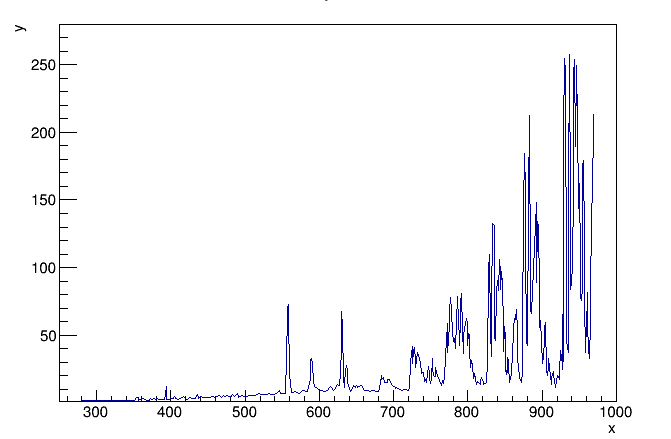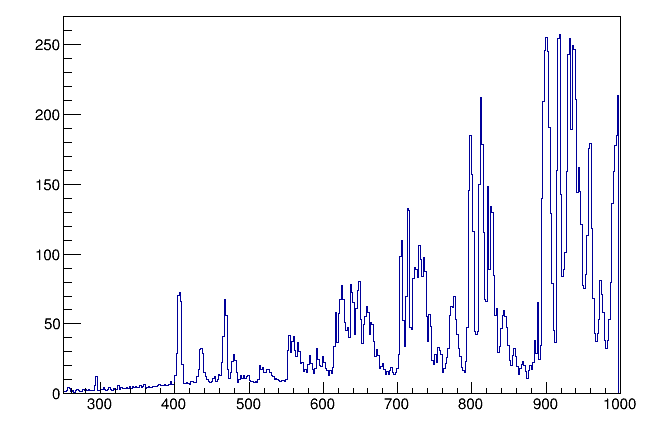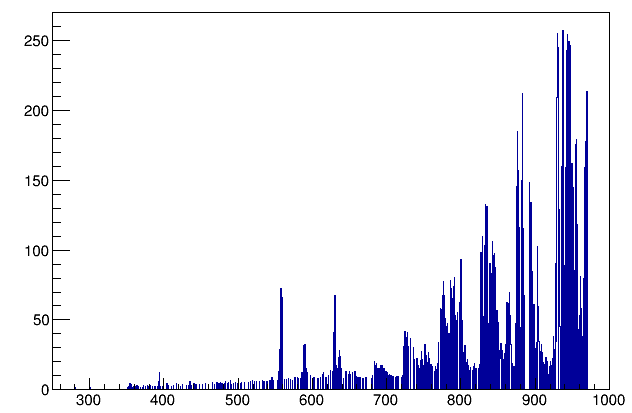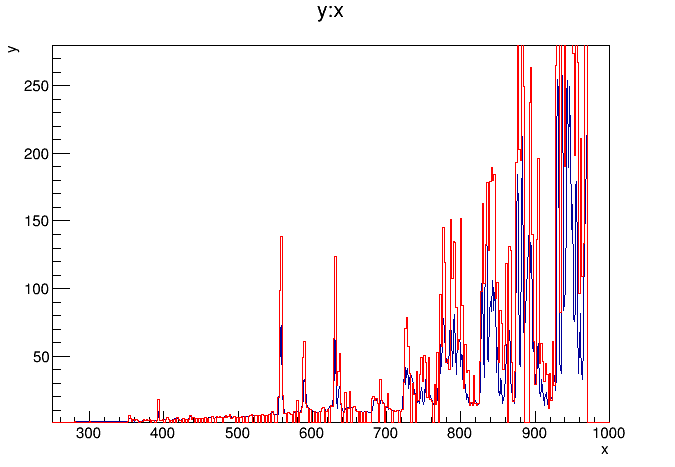# How to accurately fit a TGraph with peaks

Hi I am trying to fit TGraphs with are dominated by peaks (see example)I used the peaks.C macro from the tutorial as starting point but somehow when filling the histogram I either use a larger binning and the routine perfectly fits the histogram but the peaks are shifted
Thanksor I use such a fine binning that the fit is not describing well the TGraph since there are some empty bins.Any idea how to solve this?

I also tried to use Fill or SetBinContent but I either have the peaks to high or shifted.

So you are filling and histogram from the TGraph in order to used a peaks-C-like method (which is based on histogram) ? that’s right ?

Can you post this macro producing this shifted histogram ?

No problem …So here the macro with the data file.

I put a break in the macro in order not to launch the fitting procedure. I guess using SetBInContent is correct but then I am actually inserting the data in the wrong bin as it seems! Maybe I have to check whether the wavelength coincides with the upper and lower bin edge?

Thanks again
FitPeaks.C (4.24 KB)
NSB_detailed.txt (5.44 KB)

Thanks. I am cleaning a bit your macro to understand it. It looks a bit confusing right now…
I’ll be back…

So I reduced the useful bit of your macro to the following:

``````#include "TSpectrum"
#include "TSpectrumFit"
#include "TH1.h"
#include "TF1.h"
#include "TVirtualFitter.h"

#include <stdio.h>
#include <iostream>
#include <fstream>

Int_t npeaks = 30;
Float_t factor=1.e-6*1.e-26/1e4;//factor of 10^-6 due to micro Jy, factor of 1e-26 to conversion to J s-1 cm-2 Hz-1// in order to have cm^-2 its 10^4 cm^2
Float_t c=2.998e8; //speed of light in vaccuum in m/s reftractive index of air would be 1.000277
Float_t planck=6.62606957e-34; //planck constant to calculate enrgy of a photon with given frequency
Float_t FoV=12600.;//MAGIC FoV of 3.5 deg expressed in arsec
Float_t area=236e4;//Area of one MAGIC telescope in cm^2
//generate n peaks at random
Double_t par;

Double_t fpeaks(Double_t *x, Double_t *par) {
Double_t result = par + par*x;
for (Int_t p=0;p<npeaks;p++) {
Double_t norm  = par[3*p+2];
Double_t mean  = par[3*p+3];
Double_t sigma = par[3*p+4];
result += norm*TMath::Gaus(x,mean,sigma);
}
return result;
}

void FitPeaks(TString file,Int_t np=40){

par = 0.8;
par = -0.6/1000;

gStyle->SetOptStat(0);
Double_t x,y;
TNtupleD* data = new TNtupleD("data", "data", "x:y");

TCanvas *ntup=new TCanvas("ntuple", "ntuple");
data->Draw("y:x","","l");
TF1 *fbg=new TF1("fbg","1.42766e-2*x",400,1000);

Int_t  n = data->GetEntries();
Float_t nu;
Double_t e;
cout<<"Spectrum contains "<<n<<" points"<<endl;
data->GetEntry(0);
Float_t xmin=x;
data->GetEntry(n-1);
Float_t xmax=x;

TH1F *h=new TH1F();
TH1F *h1= new TH1F("h1","h1",n,htemp->GetXaxis()->GetXmin(),htemp->GetXaxis()->GetXmax());
TH1F *h2= new TH1F("h2","h2",n,htemp->GetXaxis()->GetXmin(),htemp->GetXaxis()->GetXmax());

for (Int_t i=0;i<n;i++) {
data->GetEvent(i);
nu=c/(x*1.e-9);
e=nu*planck;
h->Fill(x,y);
h1->Fill(x,y/e*factor);
h2->Fill(x,y/e*factor*area*FoV**2);
}

TCanvas *c4=new TCanvas();
h->Draw("l");
}``````

It gives me the attached plots.

The plot on the left is produce by

``data->Draw("y:x","","l");``

and the empty plot on the right by

``   h->Draw("l");``

So my question is, what are you trying to do there ? I do not see any TGraph (as mentioned in the title of the post). After drawing the ntuple you have already the histogram tempt containing the peaks. You can use it as the input to a peaks.C-like macro …

Hi! I modified the macro before sending and actually erased the definition of “h”

TH1F *h=new TH1F();
should be

TH1F *h= new TH1F(“h”,“h”,n,htemp->GetXaxis()->GetXmin(),htemp->GetXaxis()->GetXmax());

[quote=“couet”]So I reduced the useful bit of your macro to the following:

``````#include "TSpectrum"
#include "TSpectrumFit"
#include "TH1.h"
#include "TF1.h"
#include "TVirtualFitter.h"

#include <stdio.h>
#include <iostream>
#include <fstream>

Int_t npeaks = 30;
Float_t factor=1.e-6*1.e-26/1e4;//factor of 10^-6 due to micro Jy, factor of 1e-26 to conversion to J s-1 cm-2 Hz-1// in order to have cm^-2 its 10^4 cm^2
Float_t c=2.998e8; //speed of light in vaccuum in m/s reftractive index of air would be 1.000277
Float_t planck=6.62606957e-34; //planck constant to calculate enrgy of a photon with given frequency
Float_t FoV=12600.;//MAGIC FoV of 3.5 deg expressed in arsec
Float_t area=236e4;//Area of one MAGIC telescope in cm^2
//generate n peaks at random
Double_t par;

Double_t fpeaks(Double_t *x, Double_t *par) {
Double_t result = par + par*x;
for (Int_t p=0;p<npeaks;p++) {
Double_t norm  = par[3*p+2];
Double_t mean  = par[3*p+3];
Double_t sigma = par[3*p+4];
result += norm*TMath::Gaus(x,mean,sigma);
}
return result;
}

void FitPeaks(TString file,Int_t np=40){

par = 0.8;
par = -0.6/1000;

gStyle->SetOptStat(0);
Double_t x,y;
TNtupleD* data = new TNtupleD("data", "data", "x:y");

TCanvas *ntup=new TCanvas("ntuple", "ntuple");
data->Draw("y:x","","l");
TF1 *fbg=new TF1("fbg","1.42766e-2*x",400,1000);

Int_t  n = data->GetEntries();
Float_t nu;
Double_t e;
cout<<"Spectrum contains "<<n<<" points"<<endl;
data->GetEntry(0);
Float_t xmin=x;
data->GetEntry(n-1);
Float_t xmax=x;

TH1F *h=new TH1F();
TH1F *h1= new TH1F("h1","h1",n,htemp->GetXaxis()->GetXmin(),htemp->GetXaxis()->GetXmax());
TH1F *h2= new TH1F("h2","h2",n,htemp->GetXaxis()->GetXmin(),htemp->GetXaxis()->GetXmax());

for (Int_t i=0;i<n;i++) {
data->GetEvent(i);
nu=c/(x*1.e-9);
e=nu*planck;
h->Fill(x,y);
h1->Fill(x,y/e*factor);
h2->Fill(x,y/e*factor*area*FoV**2);
}

TCanvas *c4=new TCanvas();
h->Draw("l");
}``````

It gives me the attached plots.

The plot on the left is produce by

``data->Draw("y:x","","l");``

and the empty plot on the right by

``   h->Draw("l");``

So my question is, what are you trying to do there ? I do not see any TGraph (as mentioned in the title of the post). After drawing the ntuple you have already the histogram tempt containing the peaks. You can use it as the input to a peaks.C-like macro …[/quote]

So you macro can be reduced to:

``````void FitPeaks()
{
gStyle->SetOptStat(0);
Double_t x,y;
TNtupleD* data = new TNtupleD("data", "data", "x:y");

data->Draw("y:x","","l");

Int_t  n = data->GetEntries();

data->GetEntry(0);
Float_t xmin=x;

data->GetEntry(n-1);
Float_t xmax=x;

TH1F *h= new TH1F("h","h",n,htemp->GetXaxis()->GetXmin(),htemp->GetXaxis()->GetXmax());

for (Int_t i=0;i<n;i++) {
data->GetEvent(i);
h->Fill(x,y);
}

h->SetLineColor(kRed);
h->Draw("same");
}
``````

The plot I get seems correct …

Hi! I used your code and the plots are not the same. The position of the peaks is correct, but the height of the peaks is different! They are higher.yes because when you plot x:y from the ntuple it is discrete points, when you do fill the histogram, depending on number of bins, it may well be that two or more points fall in the same bin… try changing the number of bins.

I did this but then the fit to the historgam becomes really bad. I realized though that I had multiple entries for one wavlength since I retrieved the data via GraphClick which is limited in the resolution of the Graph. Plus I removed some entries that caused however a pile up in some bins. I used now a binning twice as large as the numbers of values such that each value is in one single bin. Lets see how the fit turns out to be (its 39 peaks to fit so it takes a while!)

You can also make an histogram with non equidistant bins making sur each bins correspondant to exactly one single x. But then I am not sure the peak teach will be happy with a such histogram.

Hi, I am still trying to solve this! How would I actually define different bin width?
Thanks

something like that:

``````
void FitPeaks()
{
gStyle->SetOptStat(0);
Double_t x,y;
TNtupleD* data = new TNtupleD("data", "data", "x:y");

data->Draw("y:x","","l");

Int_t  n = data->GetEntries();

Double_t xbins[n];
Int_t i;
for (i=0;i<n;i++) {
data->GetEvent(i);
xbins[i] = x;
}
TH1F *h= new TH1F("h","h",n-1,xbins);

for (i=0;i<n;i++) {
data->GetEvent(i);
h->Fill(x,y);
}

h->SetLineColor(kRed);
h->Draw("hist same");
}
``````

Hi! I managed to make fit the histogram in the end. However, it takes a lot of time to adjust the fit conditions to get an acceptable fit and there are usually 100 fir parameters to determine, so the fittin itself can take some time, and now I will have to redo this “exercise” for some more histograms which would mean again a lot of time investment. I was thinking to use a TSpline (black) instead, but the match of data points (red) is very poor. I would need the reconstruction of the Graph by a Fit or TSpline as precise as possible. Any idea?
Many thanks

HI,

When you have such sharp peaks, I don’t think the spline works very well.
Have you tried using Spectrum to find the peak positions and then fit it, like in the tutorial spectrum/peaks.C
see root.cern.ch/doc/master/peaks_8C.html

Cheers

Lorenzo

Hi Lorenzo!

Yes this is exactly what I did but it is too time consuming given the amount of peaks and the time spent to adjust the parameters such that the fit routine finds all relevant peaks. No alternative?

Thanks

Hi,

If you want to have a good result, the only way is to perform a fit. You can speed-up the fit by choosing appropriate initial parameters.

Lorenzo